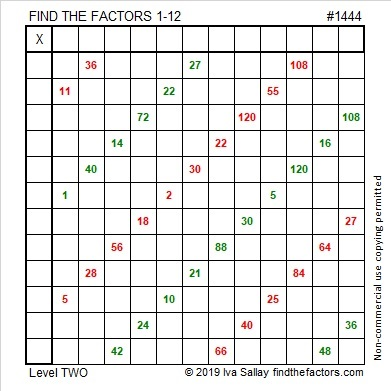# 1444 Christmas Wrapping Paper

A fourth of the products in this multiplication table puzzle are already there just because I wanted the puzzle to have a wrapping paper pattern. Can you figure out what the factors are supposed to be and what all the other products are?• 1444 is a composite number and a perfect square.
• Prime factorization: 1444 = 2 × 2 × 19 × 19, which can be written 1444 = 2²× 19²
• 1444 has at least one exponent greater than 1 in its prime factorization so √1444 can be simplified. Taking the factor pair from the factor pair table below with the largest square number factor, we get √1444 = (√4)(√361) = (2)(19) = 38
• The exponents in the prime factorization are 2 and 2. Adding one to each exponent and multiplying we get (2 + 1)(2 + 1) = 3 × 3 = 9. Therefore 1444 has exactly 9 factors.
• The factors of 1444 are outlined with their factor pair partners in the graphic below.Square number 1444 looks a lot like another square number, 144.
If we keep adding 4’s to the end, will we continue to get square numbers?
No.

However, in different bases, 1444 looks like several other square numbers:
It’s 484 in BASE 18,
400 in BASE 19,
169 in BASE 35,
144 in BASE 36,
121 in BASE 37, and
100 in BASE 38.

This site uses Akismet to reduce spam. Learn how your comment data is processed.